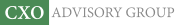Objective research to aid investing decisions

#### Value Investing Strategy (Strategy Overview)

Allocations for December 2023 (Final)
Cash TLT LQD SPY

#### Momentum Investing Strategy (Strategy Overview)

Allocations for December 2023 (Final)
1st ETF 2nd ETF 3rd ETF

# Sensitivity of Risk Adjustment to Measurement Interval

| | Posted in: Big Ideas, Mutual/Hedge Funds, Volatility Effects

Are widely used volatility-adjusted investment performance metrics, such as Sharpe ratio, robust to different measurement intervals? In the July 2014 version of their paper entitled “The Divergence of High- and Low-Frequency Estimation: Implications for Performance Measurement”, William Kinlaw, Mark Kritzman and David Turkington examine the sensitivity of such metrics to the length of the return interval used to measure it. They consider hedge fund performance, conventionally estimated as Sharpe ratio calculated from monthly returns and annualized by multiplying by the square root of 12. They also consider mutual fund performance, usually evaluated as excess return divided by excess volatility relative to an appropriate benchmark (information ratio). Finally, they consider Sharpe ratios of risk parity strategies, which periodically rebalance portfolio asset weights according to the inverse of their return standard deviations. Using monthly and longer-interval return data over available sample periods for each case, they find that:

• Extrapolation of a short-interval Sharpe ratio to a longer interval by multiplying by the square root of the number of short‐intervals in the long interval is unreliable when short-interval return autocorrelation is non-zero. Positive autocorrelation of short-interval returns makes the standard deviation of long‐interval returns higher (and the Sharpe ratio lower) than indicated by this shortcut. Hedge funds often exhibit positive return autocorrelation, such that half of funds ranked by monthly Sharpe ratios change performance deciles (tenths) when ranked by triennial (three-year) Sharpe ratios.
• Extrapolation of volatility-adjusted performance relative to a benchmark from short to long measurement intervals is unreliable when autocorrelations and/or cross-correlations of returns are non-zero. 44% of mutual funds ranked by monthly information ratios change performance deciles when ranked by triennial information ratios.
• During 1929 through 2010, a monthly rebalanced risk parity strategy applied to U.S. stocks and U.S. Treasury bonds has a higher (lower) Sharpe ratio than a monthly rebalanced 60%-40% stocks-bonds strategy for returns measured at intervals of less (more) than 40 months. Results from a more complex portfolio during 1976 through 2010 are similar.
• Evidence suggests that high-frequency variation in discount rates and low-frequency variation in cash flows drive the difference in behaviors of short-term and long-term return volatilities.

In summary, evidence suggests that volatility-adjusted performance metrics such as Sharpe ratio and information vary with the length of the measurement interval due to autocorrelation and cross-correlation effects.

In other words, investors may want to choose the longest feasible return measurement interval within their investing horizon to get the best estimate of risk-adjusted performance.

Cautions regarding findings include:

• Multi-year return measurement intervals are infeasible for many assets/strategies due to insufficiently long sample periods.
• Widely used investment performance metrics also assume that asset returns have normal distributions, whereas actual distributions may exhibit skewness and kurtosis.